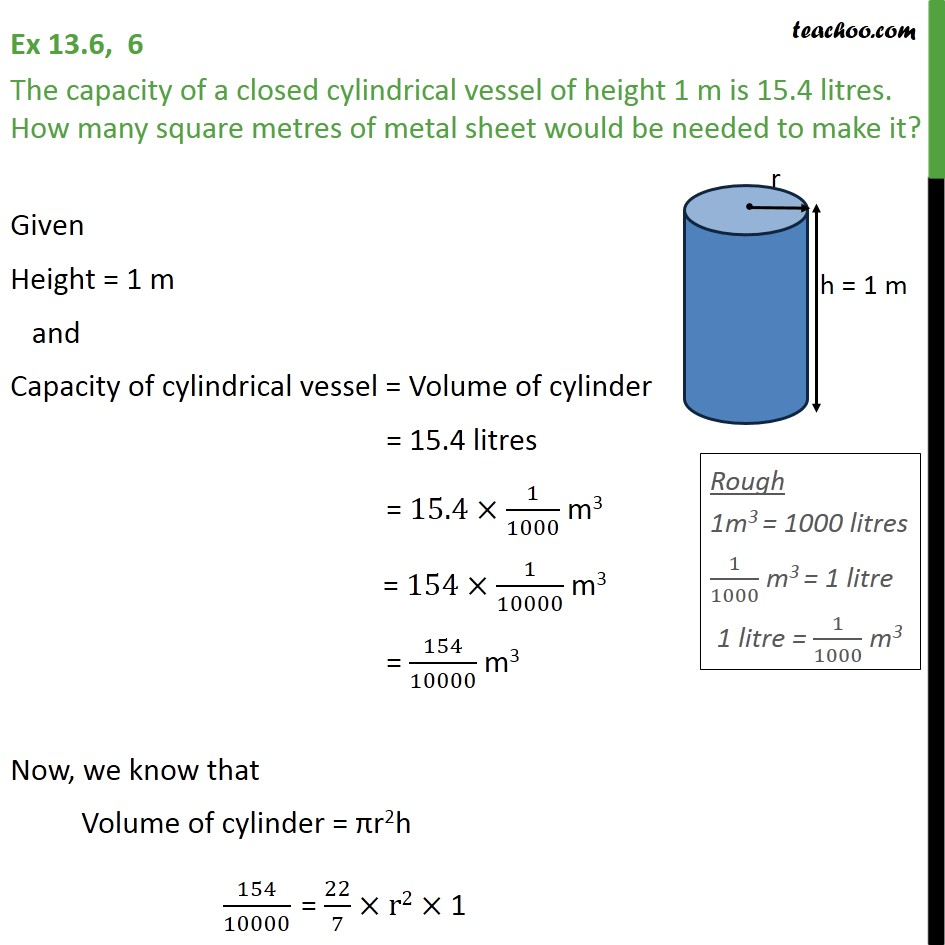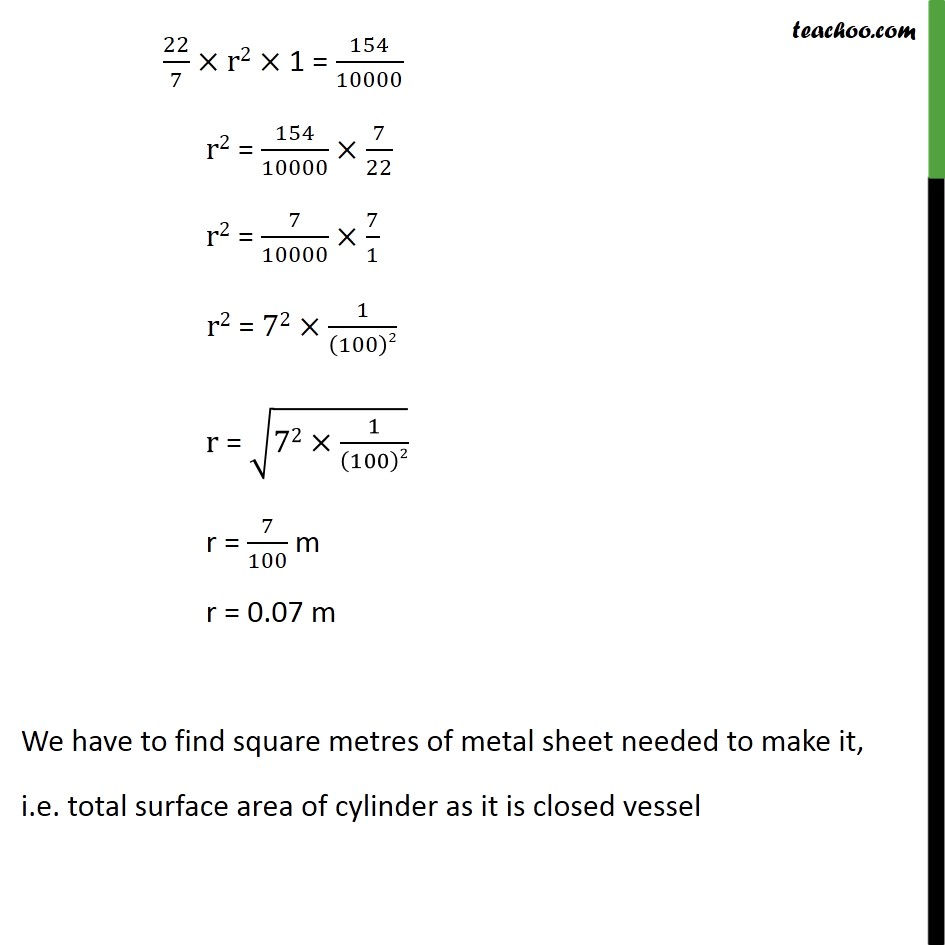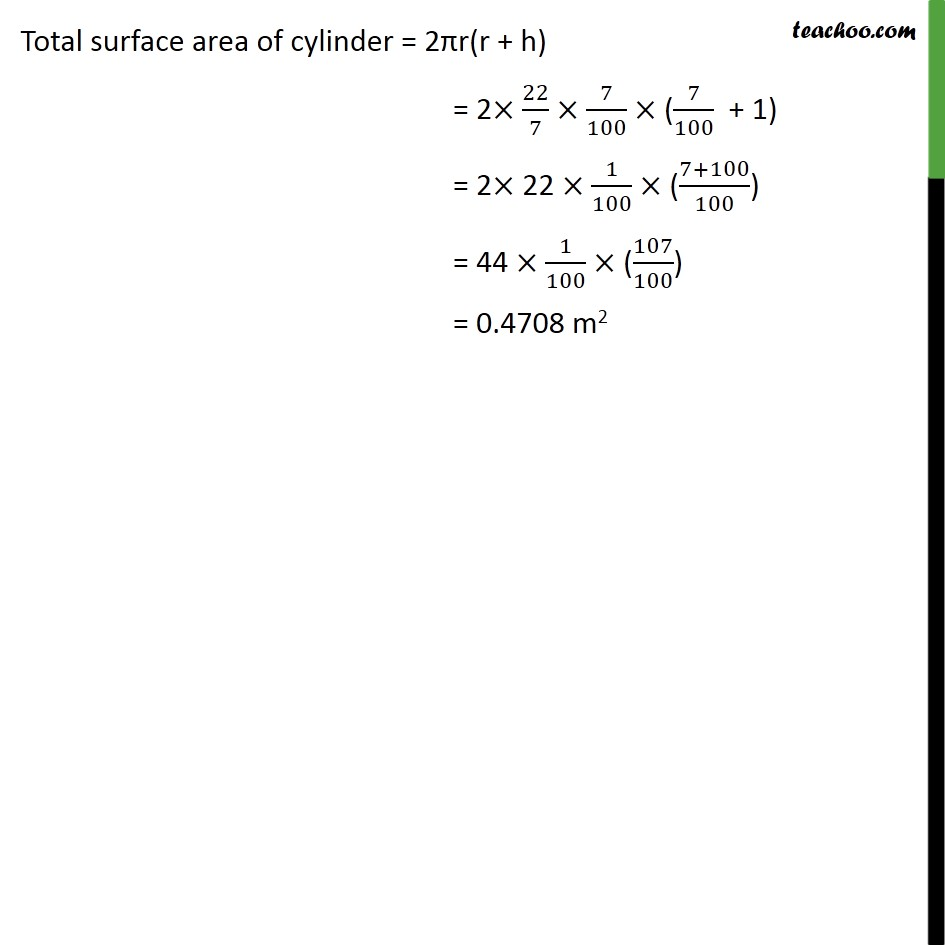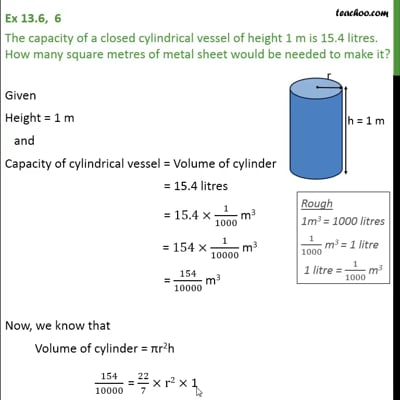Volume Of Cylinder

Chapter 13 Class 9 Surface Areas and Volumes
Concept wiseThis video is only available for Teachoo black users

Introducing your new favourite teacher - Teachoo Black, at only ₹83 per month

### Transcript

Ex 13.6, 6 The capacity of a closed cylindrical vessel of height 1 m is 15.4 litres. How many square metres of metal sheet would be needed to make it? Given Height = 1 m and Capacity of cylindrical vessel = Volume of cylinder = 15.4 litres = 15.4 1/1000 m3 = 154 1/10000 m3 = 154/10000 m3 Now, we know that Volume of cylinder = r2h 154/10000 = 22/7 r2 1 22/7 r2 1 = 154/10000 r2 = 154/10000 7/22 r2 = 7/10000 7/1 r2 = 72 1/(100)2 r = (72 1/(100)2) r = 7/100 m r = 0.07 m We have to find square metres of metal sheet needed to make it, i.e. total surface area of cylinder as it is closed vessel Total surface area of cylinder = 2 r(r + h) = 2 22/7 7/100 (7/100 + 1) = 2 22 1/100 ((7+100)/100) = 44 1/100 (107/100) = 0.4708 m2